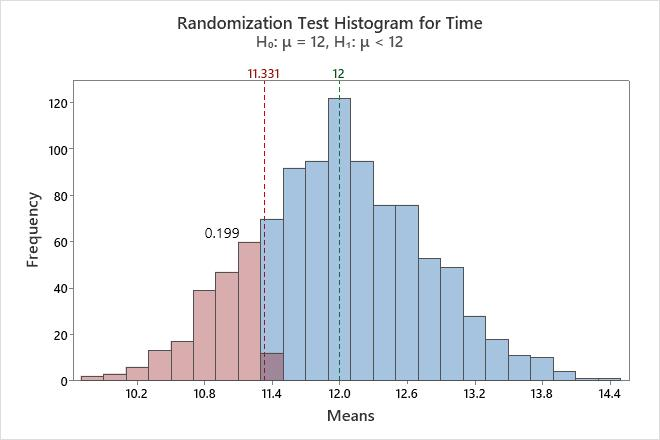# Example of Randomization test for 1-sample mean

A chemist for a pharmaceutical company wants to determine whether the mean reaction time for a newly developed antacid is less than 12 minutes.

1. Open the sample data, AntacidReactionTime.MTW.
2. Choose Calc > Resampling > Randomization Test for 1-Sample Mean.
3. In Sample, enter Time.
4. In Hypothesized mean, enter 12.
5. Click Options.
6. From Alternative hypothesis, select Mean < hypothesized value.
7. Enter 1 in Base for random number generator.
###### Note

Using Base for random number generator ensures that your results match the example.

8. Click OK in each dialog box.

## Interpret the results

The alternative hypothesis states that the mean reaction time is less than 12 minutes. Because the p-value is 0.203, which is greater than the significance level of 0.05, the chemist fails to reject the null hypothesis and cannot conclude that the mean reaction time is less than 12 minutes. The histogram shows that the bootstrap distribution appears to be normal, so the chemist can trust the results.### Randomization Test Histogram for Time

Observed Sample Variable N Mean StDev Variance Sum Minimum Median Maximum Time 16 11.331 3.115 9.702 181.300 7.700 10.050 16.000
Randomization Test Null hypothesis H₀: μ = 12 Alternative hypothesis H₁: μ < 12 Number of Resamples Mean StDev P-Value 1000 11.9783 0.7625 0.199